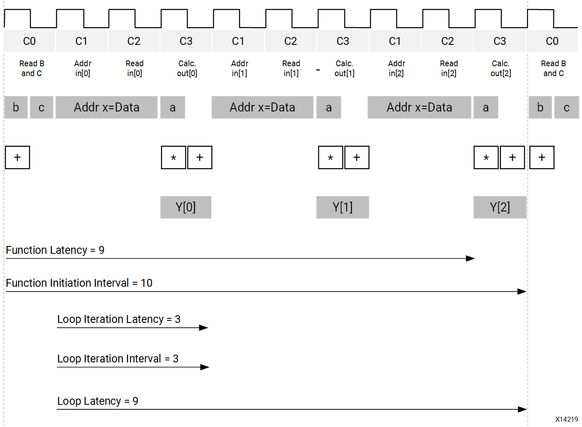Performance Metrics Example - 2021.2 English

Vitis High-Level Synthesis User Guide (UG1399)

Document ID
UG1399
ft:locale
English (United States)
Release Date
2021-12-15
Version
2021.2 English

The following figure shows the complete cycle-by-cycle execution for the code in the previous example, including the states for each clock cycle, read operations, computation operations, and write operations.

Figure 1. Latency and Initiation Interval ExampleThe following are performance metrics for this example:

• Latency: It takes the function 9 clock cycles to output all values.
Note: When the output is an array, the latency is measured to the last array value output.
• Initiation Interval (II): The II is 10, which means it takes 10 clock cycles before the function can initiate a new set of input reads and start to process the next set of input data.
Note: The time to perform one complete execution of a function is referred to as one transaction. In this example, it takes 11 clock cycles before the function can accept data for the next transaction.
• Loop iteration latency: The latency of each loop iteration is 3 clock cycles.
• Loop II: The interval is 3.
• Loop latency: The latency is 9 clock cycles.